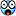# 2004 NECAS Canned Questions

## Recommended Posts

These are 20 questions from a chemistry test I do not understand. I have the answers, but no solution. If anyone could explain the solutions to me that would be great.

1. What is conjugate acid of NH4+ in the reaction: [NH4+] + [OH-] ->[H2O] + [NH3]. The answer to this: H2O

2. The freezing point of p-dichlorobenzene is 53.1 degrees Celsius; its Kf value is -7.10 oC/m. A solution of 1.25 grams of a sulfa drug in 10.0 g of p-dichlorobenzene freezes at 47.1 oC. What is the molecular weight of the sulfa drug? Answer: 148

3. A sample consisting of 25.00 mL of 0.200 M HBr is titrated with a solution of NaOH, which is 0.15 M. What is the pH of the solution after the addition of 10.00mL base? Answer: 1.00

4.The energy in kJ/mole of blue light with wavelength 400nm is: Answer:299

5. It takes 10.3 s for 1.0mL of hydrogen to effuse from a porous container. It takes 61.8 s for 1.0mL of an unknown gas to effuse under the same conditions from the porous container. The molecular mass of the gas is:72

6. The pH of a solution made from 50.0mL of 0.10M of a weak acid and 100 mL of 0.20M of its conjugate base when the pKa is 7.10. Answer: 7.70

7. The compound that forms diastereomers (optical isomers) is
https://imgur.com/a/NDeTN2q

8.  Given Ka values of 1.8*10^-5 and 6.8^10^-4 for HC2H3O2 and HF respectively, the equilibrium constant for the following reaction is: C2H3O2- + HF -> HC2H3O2 + F- Answer: 37.8

9. The solution with the lowest vapor pressure is: Answer: C
Choice:
A. water at 20 oC
B. 1M NaCl (aq) at 40 oC
C. 1M NaCl (aq) at 20 oC
D. water at 40 oC

10. Ka for hydrofluoric acid is 6.9*10^-4. What is the K for the reaction? Answer: 1.4* 10^-11
F- (aq) + H2O (l) -> HF (aq) + OH-(aq)

11. The concentration of oxygen saturated solution due to atmospheric oxygen dissolving in distilled water at room temperature is about 8.3 ppm. What would the concentration be if the concentration of oxygen in the atmosphere were
45% instead of 21%? Assume the temperature and pressure is constant. Answer: 18 ppm

12. Which indicator would you chose for a titration between a weak acid (Ka= 1.8*10^-5) and a strong base? Answer: Cresol Red (C)
A.  bromthymol blue pKa = 7.0
B. indigo carmine pKa = 13.8
C. cresol red pKa = 8.0
D. methyl red pKa = 5.1

13. A 1.000g sample of iron ore sample is converted into a solution of Fe2+ and is titrated with KMnO4. If one requires 25.0 mL of 0.0250M KMnO4, what is the percent of iron in the iron ore? The major products are Fe 3+ and Mn 2+. Answer: 17.4

14. A galvanic cell is made from a Cd strip, a Ag rod coated with AgCl and 1.0M solutions of CdCl2, AgNO3. This cell may be described as Answer: 1.242 V

Cd (s) | Cd 2+ (1.00M) || Ag+ (1.00M) | Ag(s)

The standard electrode potentials are

Cd2+ (aq) + 2e- -> Cd Eo = -0.402 C
Ag+ (aq) + e- -> Ag Eo = 0.799 V

What is the cell voltage if [Cd2+] = 0.010 M and [Ag+] = 0.50M?

15. Iodine undergoes autoxidation in basic solution. The unbalanced reaction is:

I2(s) -> IO3-(aq) + I-(aq)

When the equation is correctly balanced, the coefficient in front of I- is: Answer: 5

16. Based on the following information arrange four metals, A, B, C, and D in order of increasing ability to act as reducing agents.
I. A, B, and D do not react with 1M HCl to give H2 (g)
II. When A is added to solutions of the other metal ions, no metallic products are obtained. Answer: A
III. Metal D does not react with B n+

A. C>B>D>A
B. C>B>A>D
C. B>C>D>A
D. B>C>A>D

17. A student determines the concentration of a NaOH solution. She pipets the NaOH solution into a flask and adds two drops of indicator. Standardized acid is added from a buret until the indicator changes color. Which device may contain residual water without affecting the results of the titration? Answer: B

1. buret
3. pipet

A. 1 only
B. 2 only
C. 1 and 3 only
D. 2 and 3 only

18. The drug, ibuprofen, is shown on the right. Which region of ibuprofen is most responsible for the fact that this drug dissolves well in nonpolar cell membranes and fatty tissues?
https://imgur.com/a/PYYCt2q

##### Share on other sites

As mentions in the other forum, what is your own work in this?

##### Share on other sites

The first one is pretty close to being trivial!  H2O is the only "acid" in the equation!

##### Share on other sites

For the first question the acid concept of Brönstedt should be used.

According him acids are proton donators.

In the given equation is NH4+ / H2O the donators.

Bases are proton acceptors.

So its NH3 / OH-  for base pair.

##### Share on other sites

8 hours ago, Jake S. said:

These are 20 questions from a chemistry test I do not understand. I have the answers, but no solution. If anyone could explain the solutions to me that would be great.

What all 20 ?What subject are you studying that you do not know the answers to any of them  ?

Did you really not understand it ?

This is simply a matter of common sense, although it contains some points of chemistry worth discussing

I see that HOI and Chenbeier have done question 1 to death, it really is a chemistry question. +1 apiece.

Edited by studiot
##### Share on other sites

• 3 weeks later...

Thank you very much for sharing this knowledge

## Create an account

Register a new account